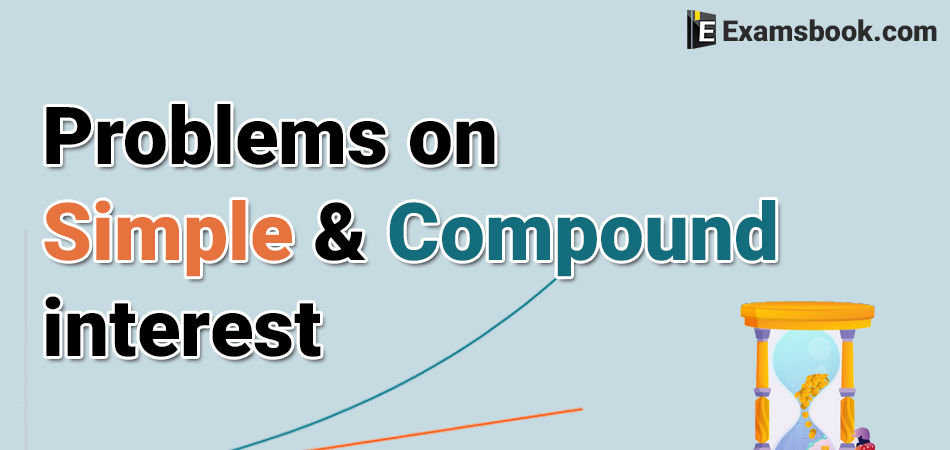• Save

# Problems on Simple Interest and Compound Interest for Bank ExamsSimple interest and compound interest questions are mostly asked in banking exams. In the simple interest questions, you have to calculate interest according to the time and rate whereas, in compound interest questions you have to add interest to the principal sum of deposit, or in other words, interest on interest.

So here I am sharing problems on simple interest and compound interest for bank exams with answers for your better preparation. You can easily score high in the competitive exams by practicing these selective questions.

As well as you can understand easily about Simple and Compound Interest Formulas that how to use formulas in these types of questions.

## Simple interest and Compound Interest Problems

### Simple interest Problems:

Q.1. What will be the ratio of simple interest earned by certain amount at the same rate of interest for 6 years and that for 9 years?

(A) 1 : 3

(B) 1 : 4

(C) 2 : 3

(E) None of these

Ans .   C

Q.2. A man took loan from a bank at the rate of 12% p.a. simple interest. After 3 years he had to pay Rs. 5400 interest only for the period. The principal amount borrowed by him was:

(A) Rs.2000

(B) Rs.10000

(C) Rs.15000

(D) Rs.20000

Ans .   C

Q.3. A certain amount earns simple interest of Rs. 1750 after 7 years. Had the interest been 2% more, how much more interest would it have earned?

(A) Rs. 35

(B) Rs. 245

(C) Rs. 350

(D)  Cannot be determined

(E) None of these

Ans .   D

Q.4. A sum of money at simple interest amounts to Rs. 815 in 3 years and to Rs. 854 in 4 years. The sum is:

(A) 650

(B) 690

(C) 698

(D) 700

Ans .   C

Q.5. A person borrows Rs. 5000 for 2 years at 4% p.a. simple interest. He immediately lends it to another person at 6p.a for 2 years. Find his gain in the transaction per year.

(A) Rs. 112.50

(B) Rs. 125

(C) Rs. 150

(D)  Rs. 167.50

Ans .   A

Q.6. In how many years will a sum of Rs.800 at 10% per annum compounded semi annually become Rs.926.10

(A) 1.5

(B) 2.5

(C) 3.5

(D) 4.5

Ans .   A

Q.7. A sum of money amounts to Rs. 9800 after 5 years and Rs. 12005 after 8 years at the same rate of simple interest. The rate of interest per annum is:

(A) 5%

(B) 8%

(C) 12%

(D)  15%

Ans .   C

Q.8. At what rate percent per annum will a sum of money double in 8 years.

(A) 12.5%

(B) 13.5%

(C) 11.5%

(D) 14.5%

Ans .   A

Q.9. What percentage of simple interest per annum did Ram pay to Shivam?

I. Ram borrowed Rs. 8000 from Shivam for four years.

II. Ram returned Rs. 8800 to Shivam at the end of two years and settled the loan.

(A) I alone sufficient while II alone not sufficient to answer

(B) II alone sufficient while I alone not sufficient to answer

(C) Either I or II alone sufficient to answer

(D)  Both I and II are not sufficient to answer

(E) Both I and II are necessary to answer

Ans .   E

Q.10. What annual instalment will discharge a debt of Rs 1092 due in 3 years at 12% simple interest?

(A) Rs.325

(B) Rs.545

(C) Rs.560

(D) Rs.550

Ans .   A

If you are facing any problems in the simple and compund interest problems with answers, ask me in the comment section without any hesitation. Visit on the next page for more practice.